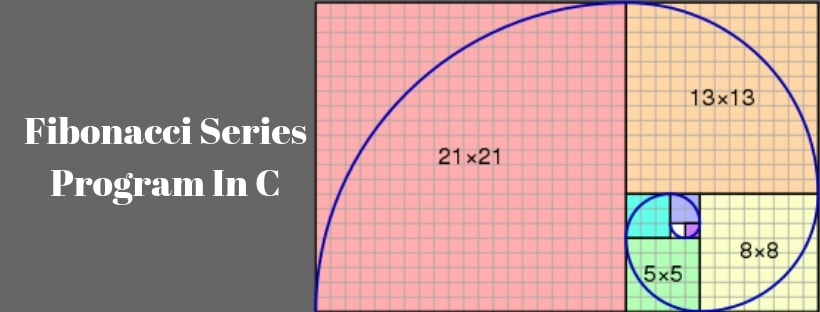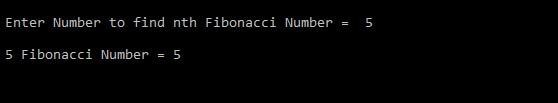# Fibonacci Series Program In CIn the Fibonacci series, each number is the sum of the two previous number. The first two numbers in the Fibonacci series are 0 and 1.

The sequence Fn of Fibonacci numbers is defined by the recurrence relation:

Fn = Fn-1 + Fn-2

with seed values

F0 = 0 and F1 = 1.

So if n =7, the Fibonacci series can look like this:

F7 = 0 1 1 2 3 5 8

### Methods to get the nth Fibonacci number:

#### Recursive way to find an nth Fibonacci number.#### Code Analysis

• If (n < 0) – check whether the given number is +ve or not. If it is TRUE, the function will return an error message.
• If (n== 0) – check whether the given number is 0 or not. If it is TRUE, the function will return Zero.
• If (Number == 1) – check the specified number is equal to 1 or not. If it is TRUE, the function will return One.
• If the number is greater than 1, then the recursive operation is performed.

Time Complexity: T(n) = T(n-1) + T(n-2) .

You can observe the recursion tree that this implementation does a lot of repeated work. So it is not a good way to find the nth Fibonacci number.

#### Optimize way to find an nth Fibonacci number

In the above code, simply we are using the concept Fn = Fn-1 + Fn-2 .
.